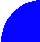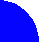"JOURNAL OF RADIOELECTRONICS" N 6, 2006 contents full text discussionNUMERICAL ANALYSIS OF WAVEGUIDES AND WAVEGUIDE ELEMENTS IN EBG STRUCTURES

# S. E. Bankov, M.D. Duplenkova Institute of Radioengineering and Electronics of RAS

Received December 15, 2006

This paper presents an efficient technique for analysis of waveguides, resonators and waveguide discontinuities in two-dimensional electromagnetic bandgap (EBG) structures. Waveguides and resonators formed by elements removed from EBG array are considered. The technique includes the following key steps. The first step is a solution of problem of electromagnetic (EM) field scattering on a single element of array. Scattered field is presented as a finite series of cylindrical harmonics with coefficients depending on incident field. The second step is an introduction of a compensating source (CS) and excitation of a homogeneous EBG structure by the source. CS produces EM field in the same form of sum of cylindrical harmonics but with fixed coefficients. The last third step is analysis of EM field in waveguide or resonance structure inside EBG array. Elements forming the structure are not removed from array but instead of it CSs with unknown coefficients are placed in the elements. These coefficients are defined under condition that total field produced by the element (scattered field plus field induced by a CS) is equal to zero that is equivalent to removal of the element from array. These conditions formulated for all of removed elements give one a system of linear algebraic equations (SLAE) relatively mentioned coefficients that is simply solved numerically or analytically. Resonator, infinite waveguide, waveguide with lumped discontinuity and waveguide bends are considered in the paper.